# Analytical Functions in Oracle

## Analytical Functions in Oracle with Examples

In this article, I am going to discuss Analytical Functions in Oracle with examples. Please read our previous article where we discussed Inline View Subquery in Oracle with Examples. At the end of this article, you will understand everything about the Ranking Functions in Oracle with Examples.

##### Analytical Functions in Oracle

Oracle supports the following three types of analytical functions,

These analytical functions are automatically generated ranking numbers for each row-wise (or) group of rows-wise. These are also called “Ranking Functions” in Oracle. For better understanding, please have a look at the below image.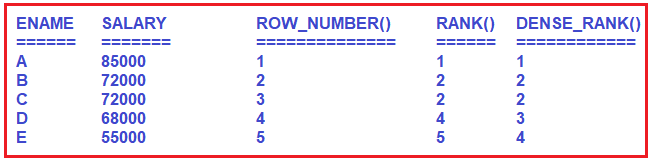As you can see in the above image, the ROW_NUMBER() function will assign a different rank number to the same value whereas the RANK(), DENSE_RANK() function will assign the same rank number to the same value. But Rank() function will skip the next rank number in the order whereas DENSE_RANK() function will not skip the next rank number in the order.

Syntax: ANALYTICAL FUNCTION NAME() OVER( [ PARTITION BY <COLUMN NAME> ] ORDER BY <COLUMN NAME> [ASC / DESC ])

Here, the PARTITION BY clause is optional and the ORDER BY clause is mandatory. So, let us first understand the OVER clause in Oracle, and then we will see the Analytical Functions examples.

##### OVER Clause in Oracle:

The OVER clause in Oracle is used with the PARTITION BY clause to break the data into partitions.

<FUNCTION> OVER( [ PARTITION BY <COLUMN NAME> ] ORDER BY <COLUMN NAME> [ASC / DESC ])

Here, the specified function is going to operate for each partition. Please have a look at the below example for a better understanding. Let say we have three departments (HR, IT, Payroll).

COUNT (Department) OVER (PARTITION BY Department)

In the above example, the data will be partitioned by Department i.e. there will be three partitions (IT, HR, and Payroll), and then the COUNT() function will be applied to each partition. Here, you can use a wide range of built-in functions such as COUNT(), SUM(), MAX(), ROW_NUMBER(), RANK(), DENSE_RANK(), AVG(), MIN(), etc. If this is not clear at the moment, then don’t worry, we will see examples of each function and try to understand the need and power of the OVER Clause in Oracle.

##### Example: OVER clause in Oracle

We are going to use the following Employees table to understand the need and use of the Over clause in Oracle.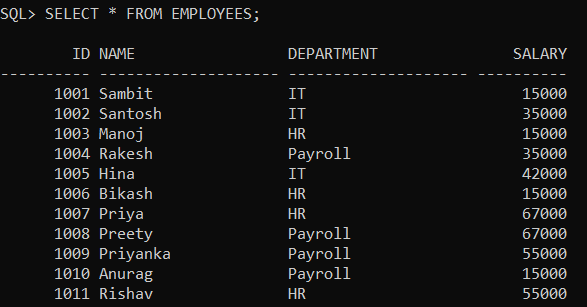Please use the following SQL Script to create and populate the Employees table with the required sample data.

```CREATE TABLE Employees
(
ID INT PRIMARY KEY,
Name VARCHAR2(20),
Department VARCHAR(20),
Salary int
);

INSERT INTO Employees Values (1001, 'Sambit', 'IT', 15000);
INSERT INTO Employees Values (1002, 'Santosh', 'IT', 35000);
INSERT INTO Employees Values (1003, 'Manoj', 'HR', 15000);
INSERT INTO Employees Values (1004, 'Rakesh', 'Payroll', 35000);
INSERT INTO Employees Values (1005, 'Hina', 'IT', 42000);
INSERT INTO Employees Values (1006, 'Bikash', 'HR', 15000);
INSERT INTO Employees Values (1007, 'Priya', 'HR', 67000);
INSERT INTO Employees Values (1008, 'Preety', 'Payroll', 67000);
INSERT INTO Employees Values (1009, 'Priyanka', 'Payroll', 55000);
INSERT INTO Employees Values (1010, 'Anurag', 'Payroll', 15000);
INSERT INTO Employees Values (1011, 'Rishav', 'HR', 55000);
```
##### Example:

Our business requirement is to generate a report that should display the total number of employees in each department. Along with the total number of employees in each department, we also need to display the Total Salary, Average Salary, Minimum Salary, and Maximum Salary of each department. That means, our business requirement is to generate a report as shown in the below image.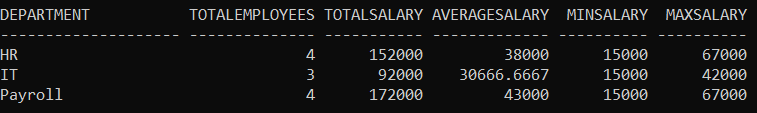We can easily achieve the above output by using the Oracle Aggregate function along with the GROUP BY clause as shown in the below query.

```SELECT  Department,
COUNT(*) AS TotalEmployees,
SUM(Salary) AS TotalSalary,
AVG(Salary) AS AverageSalary,
MIN(Salary) AS MinSalary,
MAX(Salary) AS MaxSalary
FROM Employees
GROUP BY Department;
```
##### Example:

Now our business requirement changes. Now we also want to show the non-aggregated values (Name and Salary) in the report along with the aggregated values as shown in the below image.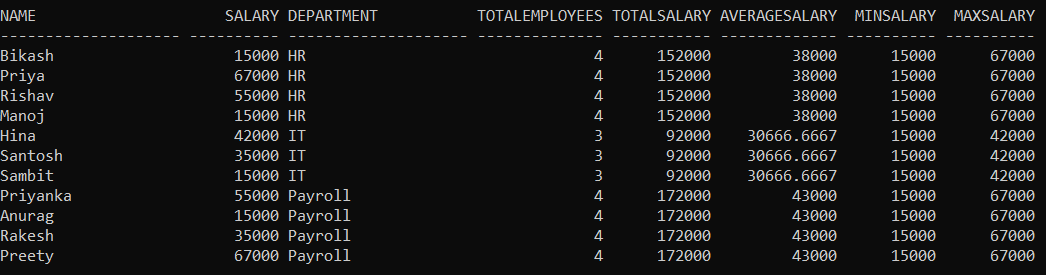Now, you may be intended to use the following SQL query by adding the Salary, Name column in the select clause. But this is not going to work.

```SELECT Name, Salary, Department,
COUNT(*) AS TotalEmployees,
SUM(Salary) AS TotalSalary,
AVG(Salary) AS AverageSalary,
MIN(Salary) AS MinSalary,
MAX(Salary) AS MaxSalary
FROM Employees
GROUP BY Department;
```

When you execute the above query, you will get the following error. This is because Name and Salary are not present in the group by clause in the query.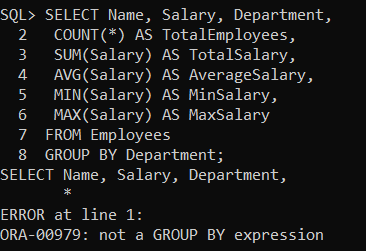In order to get the desired output is by using the OVER clause combined with the PARTITION BY clause as shown in the below code.

```SELECT Name, Salary, Department,
COUNT(Department) OVER(PARTITION BY Department) AS TotalEmployees,
SUM(Salary) OVER(PARTITION BY Department) AS TotalSalary,
AVG(Salary) OVER(PARTITION BY Department) AS AverageSalary,
MIN(Salary) OVER(PARTITION BY Department) AS MinSalary,
MAX(Salary) OVER(PARTITION BY Department) AS MaxSalary
FROM Employees;
```

Once you execute the above query, you will get the output as expected.

##### Analytical Functions Without Partition by Clause in Oracle:

In the below SQL query, we are using the Ranking Functions without Partition by Clause.

```SELECT Name, Salary,
ROW_NUMBER() OVER(ORDER BY Salary DESC) ROW_NUMBER,
RANK() OVER(ORDER BY Salary DESC) RANK,
DENSE_RANK() OVER(ORDER BY Salary DESC) DENSE_RANK
FROM Employees;```

When you execute the above query, you will get the following output.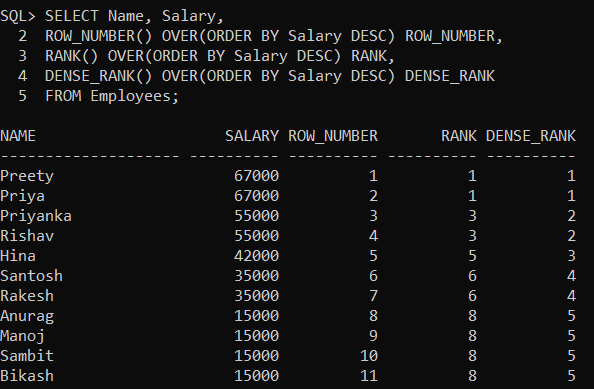##### Analytical Functions With Partition by Clause in Oracle:

In the below SQL query, we are using the Ranking Functions with Partition by Clause.

```SELECT NAME, SALARY,
ROW_NUMBER() OVER(PARTITION BY DEPARTMENT ORDER BY SALARY DESC) ROW_NUMBER,
RANK() OVER(PARTITION BY DEPARTMENT  ORDER BY SALARY DESC) RANK,
DENSE_RANK() OVER(PARTITION BY DEPARTMENT ORDER BY SALARY DESC) DENSE_RANK
FROM EMPLOYEES;
```

When you execute the above query, you will get the following output.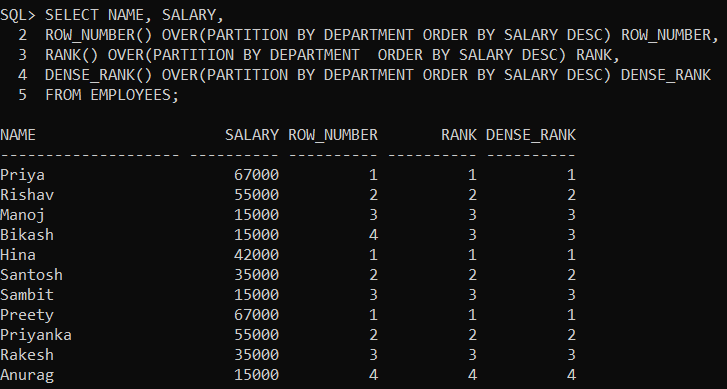##### Example:

WAQ to display 3rd highest salary employee details from Employees table in each department wise by using DENSE_RANK() function along with inline view?
Solution:
SELECT * FROM (SELECT NAME, SALARY, DEPARTMENT,
DENSE_RANK() OVER (PARTITION BY DEPARTMENT ORDER BY SALARY DESC) R FROM EMPLOYEES) WHERE R=3;
Output: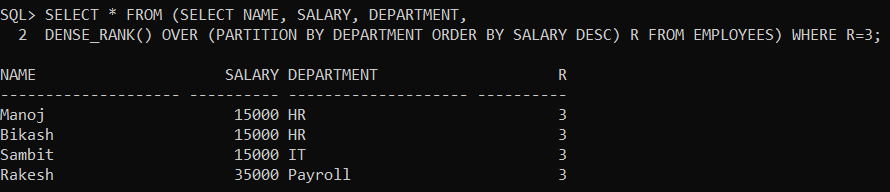In the next article, I am going to discuss the ROW_NUMBER Function in Oracle with examples. Here, in this article, I try to explain the need and use of Analytical Functions in Oracle with Examples. I hope you enjoy this article.